Drop a Query

# Statisctics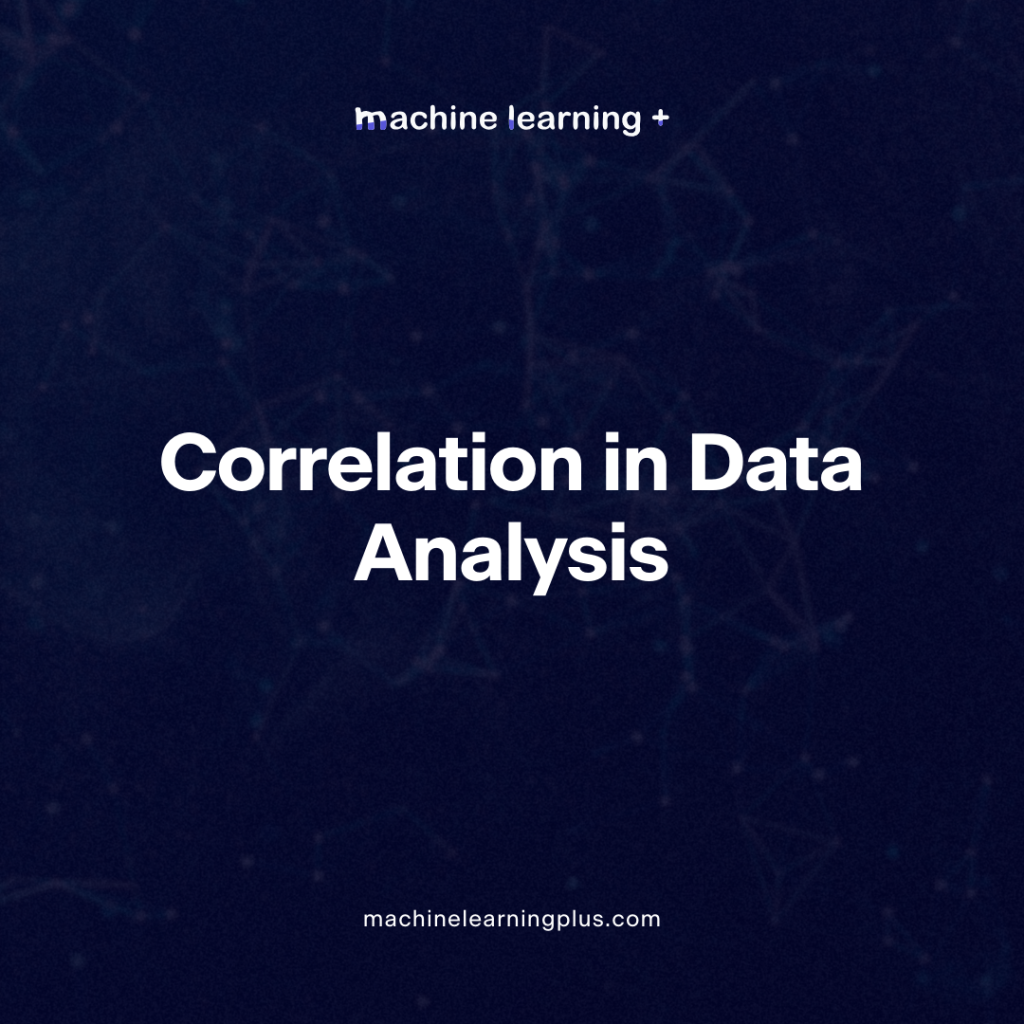## Correlation – Connecting the Dots, the Role of Correlation in Data Analysis

Correlation is a fundamental concept in statistics and data science. It quantifies the degree to which two variables are related. But what does this mean, and how can we use it to our advantage in real-world scenarios? Let’s dive deep into understanding correlation, how to measure it, and its practical implications. In this Blog post …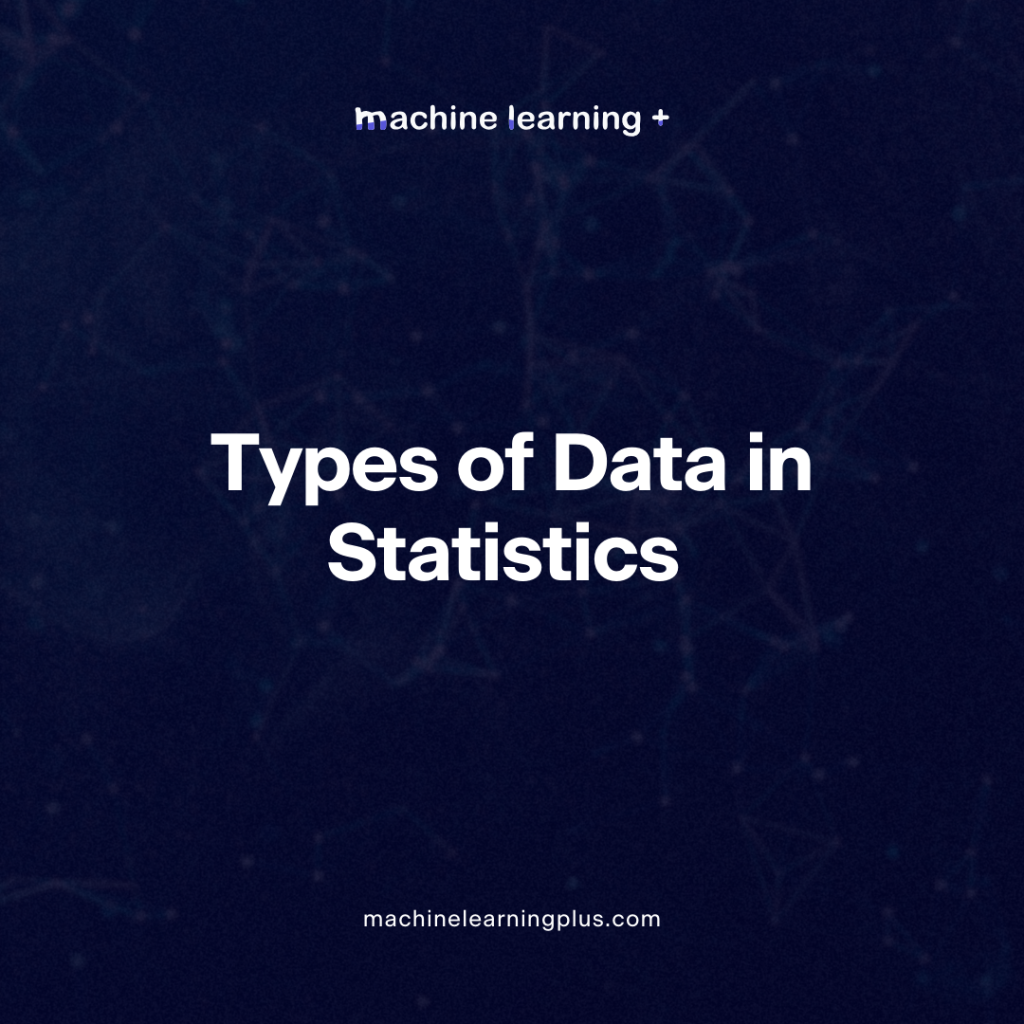## Types of Data in Statistics – A Comprehensive Guide

Statistics is a domain that revolves around the collection, analysis, interpretation, presentation, and organization of data. To appropriately utilize statistical methods and produce meaningful results, understanding the types of data is crucial. In this Blog post we will learn Qualitative Data (Categorical Data) 1.1. Nominal Data: 1.2. Ordinal Data: Quantitative Data (Numerical Data) 2.1. Discrete …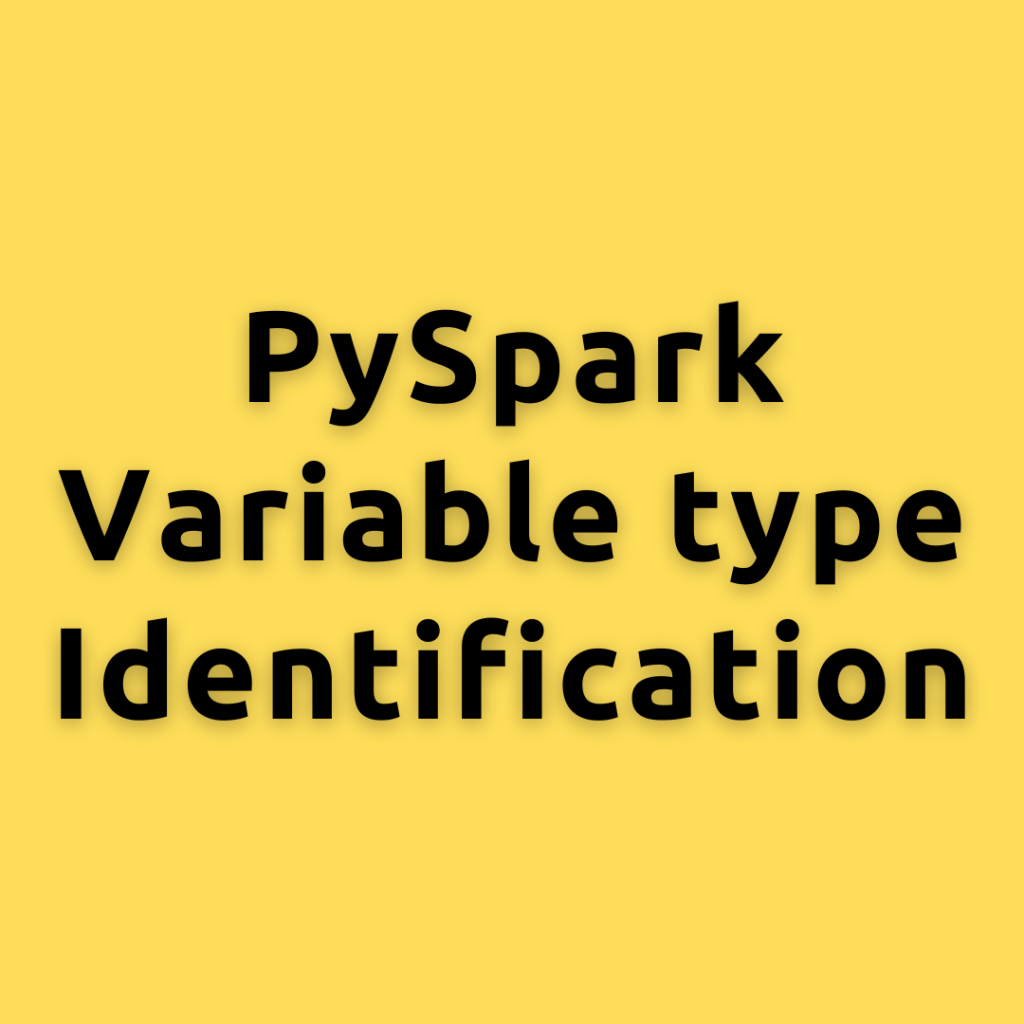## PySpark Variable type Identification – A Comprehensive Guide to Identifying Discrete, Categorical, and Continuous Variables in Data

Let’s Explore what are discrete, categorical, and continuous variables, their identification techniques, and their importance in machine learning and statistical modeling. Data preprocessing is a critical step in machine learning and statistical modeling. Before diving into model building, it is essential to understand and identify the types of variables present in the dataset. Furthermore, I …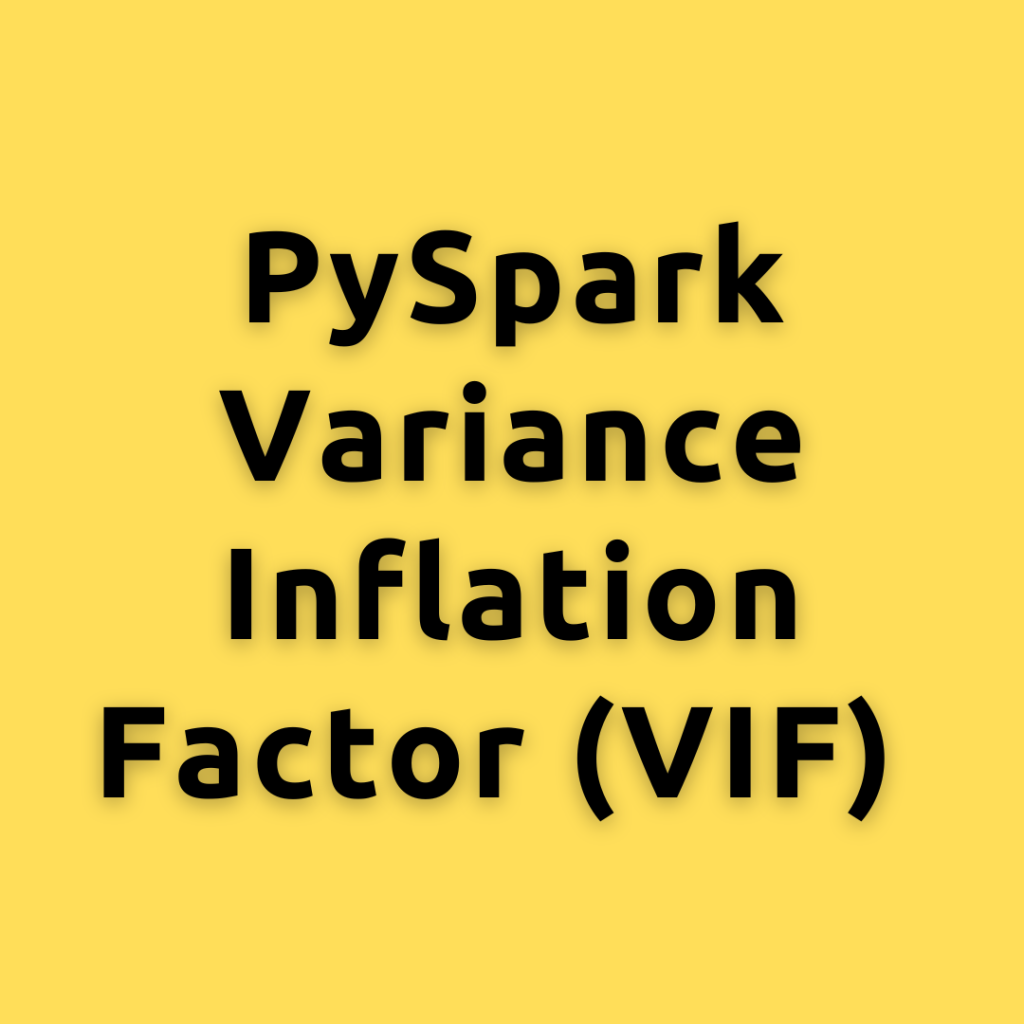## PySpark Variance Inflation Factor (VIF) – Understanding of VIF and how it can help you improve your regression models.

VIF concept is critical for understanding multicollinearity in regression models, let’s break down the concept into simple terms, explain how to calculate VIF, and discuss its practical uses What is Variance Inflation Factor (VIF)? VIF is a measure that helps us understand the extent of multicollinearity in a multiple regression model. Multicollinearity occurs when two …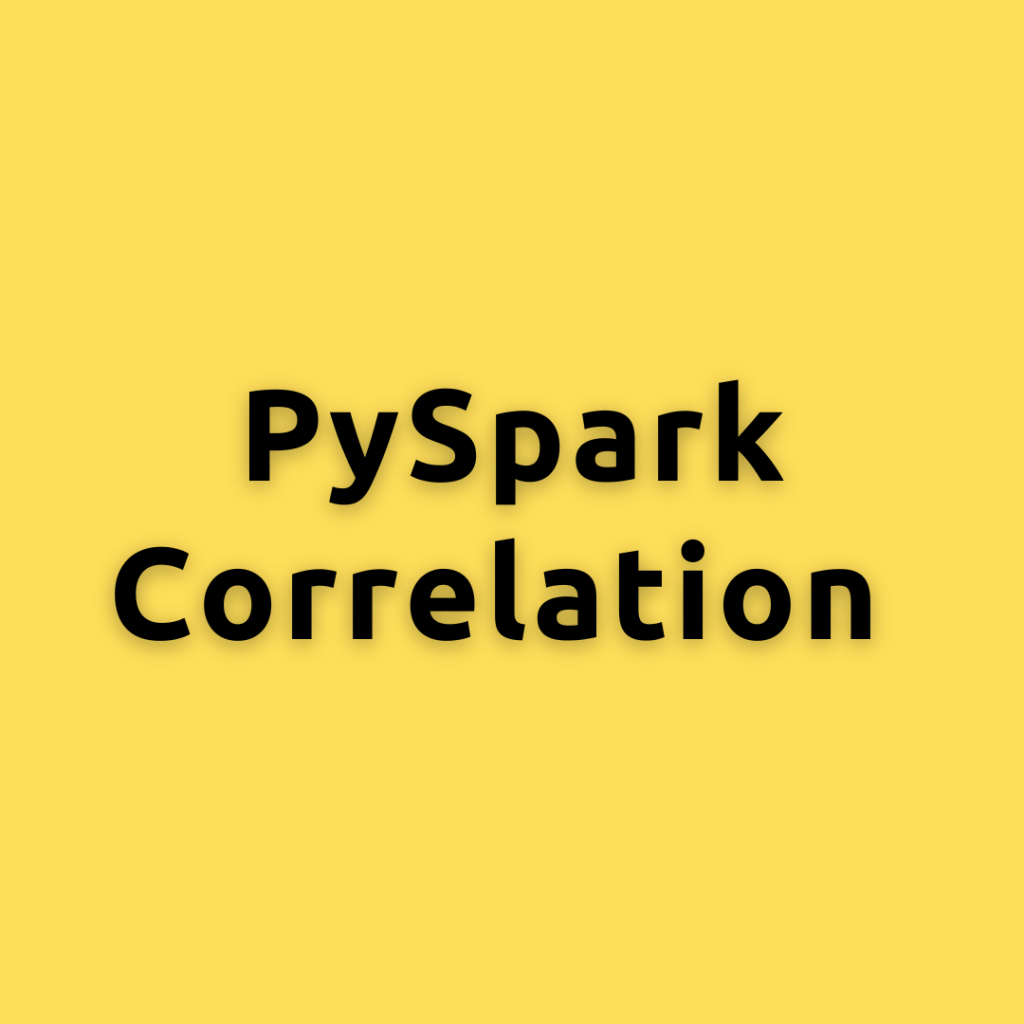## PySpark Correlation – Understanding Correlation a Deep Dive with PySpark

Lets dive into the concept of correlation, explore how to calculate it using PySpark in different ways, and discuss its applications in statistics and machine learning. In the data-driven world we live in, correlation is a key concept that is frequently used in various fields, including statistics and machine learning. Understanding the relationship between variables …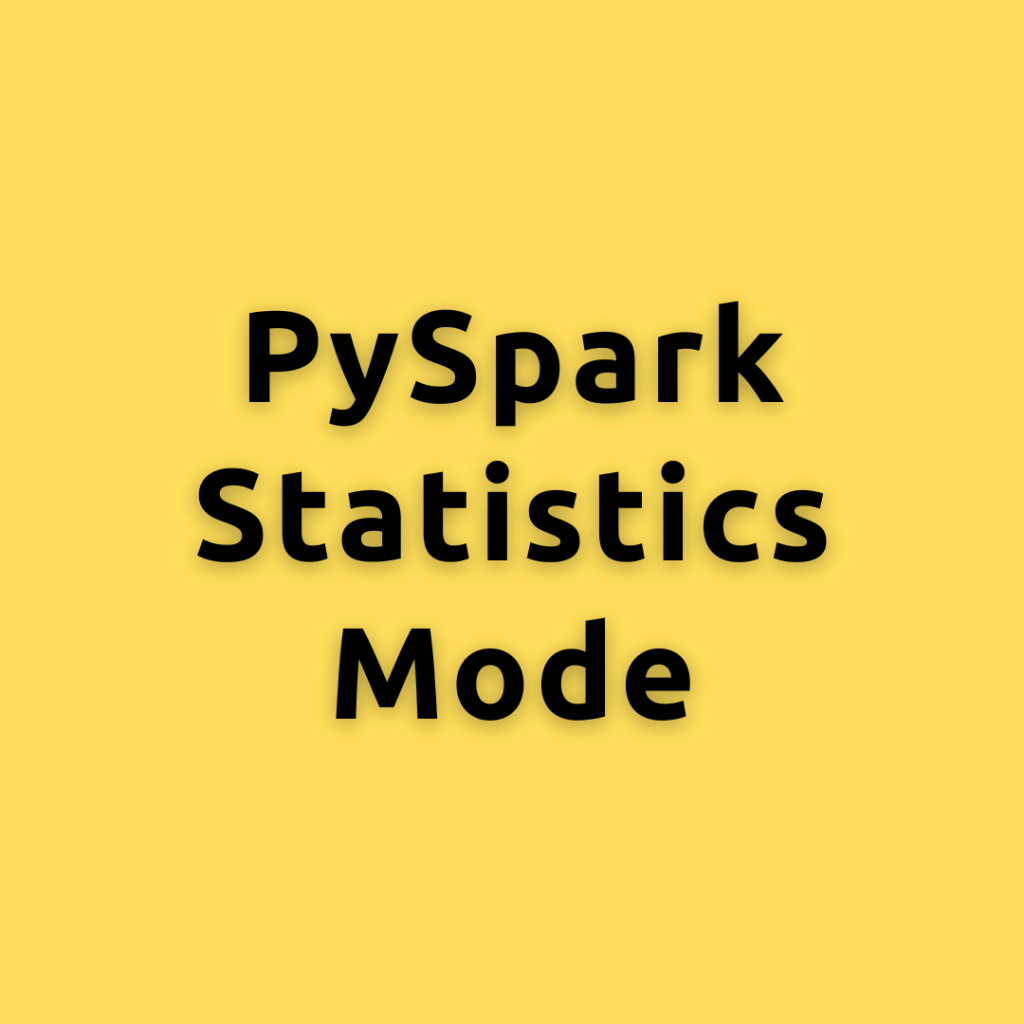## PySpark Statistics Mode – Calculating the Mode in PySpark a Comprehensive Guide for Everyone

Lets explore different ways of calculating the Mode using PySpark, helping you become an expert Mode is the value that appears most frequently in a dataset. It is a measure of central tendency, similar to mean and median, but focuses on the most common value(s) in the data. Mode can be applied to both numerical …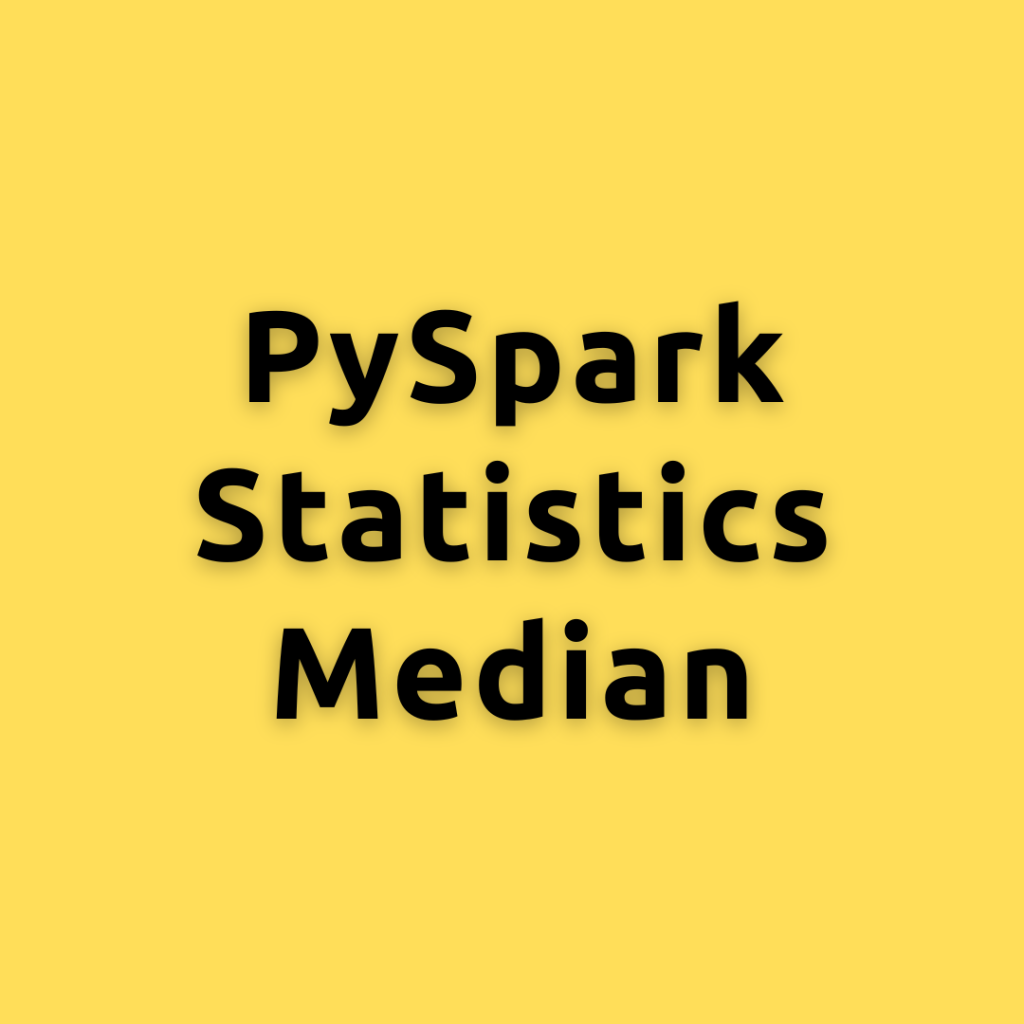## PySpark Statistics Median – Calculating the Median in PySpark a Comprehensive Guide for Everyone

Lets explore different ways of calculating the Median using PySpark, helping you become an expert As data continues to grow exponentially, efficient data processing becomes critical for extracting meaningful insights. PySpark, an Apache Spark library, enables large-scale data processing in Python. How to Calcualte Median? The median is a measure of central tendency that represents …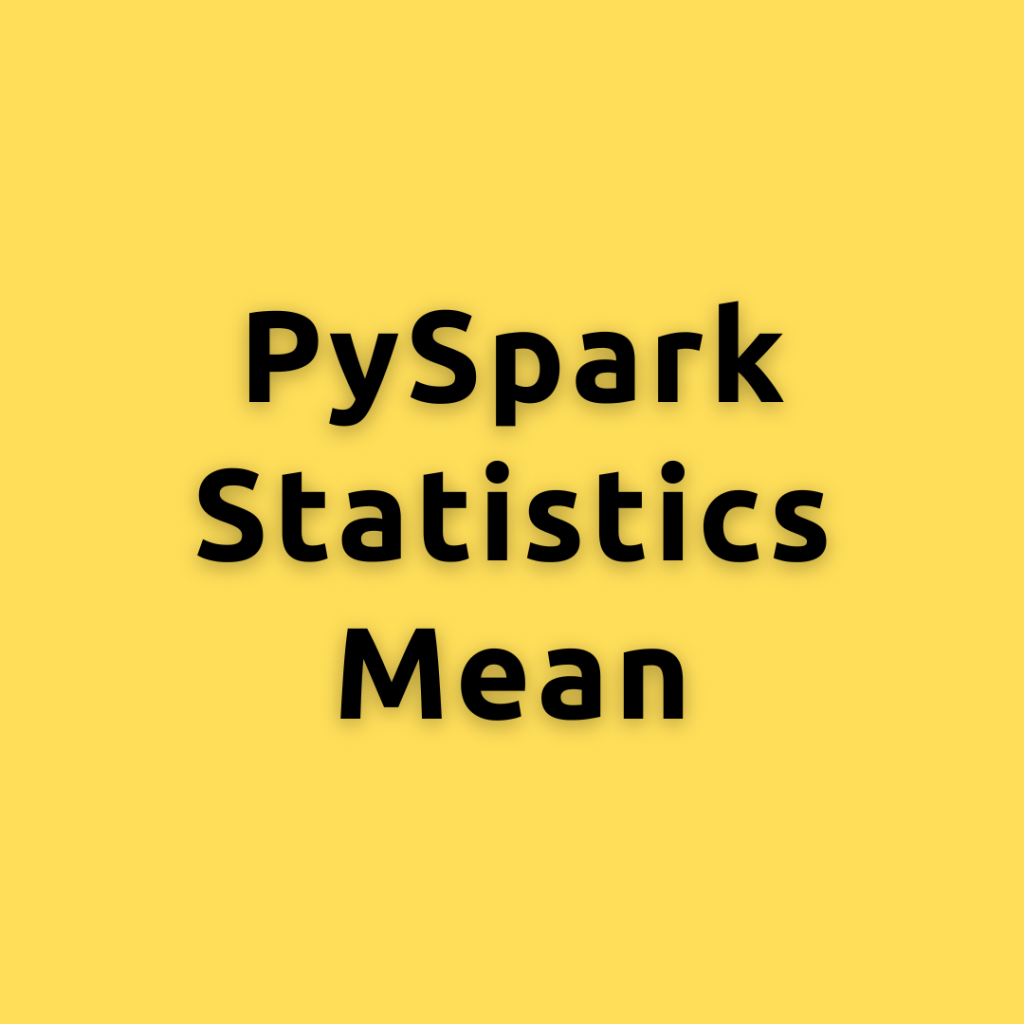## PySpark Statistics Mean – Calculating the Mean Using PySpark a Comprehensive Guide for Everyone

Lets explore different ways of calculating the mean using PySpark, helping you become an expert in no time As data continues to grow exponentially, efficient data processing becomes critical for extracting meaningful insights. PySpark, an Apache Spark library, enables large-scale data processing in Python. Concept of Mean: The mean, also known as the average, is …Course Preview

## Machine Learning A-Z™: Hands-On Python & R In Data Science

### Free Sample Videos:#### Machine Learning A-Z™: Hands-On Python & R In Data Science#### Machine Learning A-Z™: Hands-On Python & R In Data Science#### Machine Learning A-Z™: Hands-On Python & R In Data Science#### Machine Learning A-Z™: Hands-On Python & R In Data Science